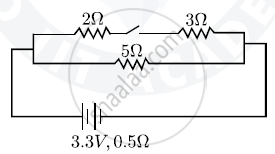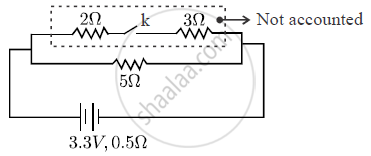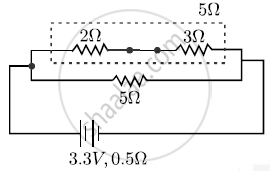# The diagram above shows a circuit with the key k open. Calculate : (i) the resistance of the circuit when the key k is open. (ii) the current drawn from the cell when the key k is open - Physics

Short NoteThe diagram above shows a circuit with the key k open. Calculate :
(i) the resistance of the circuit when the key k is open.
(ii) the current drawn from the cell when the key k is open.
(iii) the resistance of the circuit when the key k is closed.
(iv) the current drawn from the cell when the key k is closed.

#### Solution

(i) when k is openReq = 5Ω + 0.5 Ω = 5.5Ω

(ii) i = v/(R_(eq))|_("case k open")

=(3.3v)/(5.5 Ω ) = 3/5 =0.6 A

(iii) when k is closed,R_(eq) =(R_1R_2)/(R_1 + R_2) = (5xx5)/(5+5) = 2.5 Ω

R_(Total) = 2.5 Ω + 0.5 Ω = 3.0 Ω

(iv) i =v/(R_T) = 3.3/3.0 = 1.1A

Concept: Electrical Power
Is there an error in this question or solution?